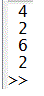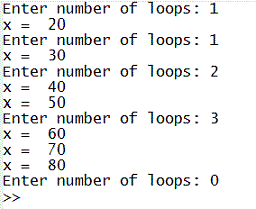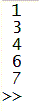# Break statement and Continue in  Matlab

1.- Break

2.- Continue

3.- Video Summary

### 1.- The break Statement

The break statement lets you exit early from a for or while loop. In nested
loops, break exits from the innermost loop only. It is part of the flow control in programming.

Example 1:

% Let's say that you have an array that you want to explore
y = [-2 -4 0 -4 3 7];

% You're gonna test each element for a special condition
for i = 1 : length(y)

% Test for a greater-than-zero value
if y(i) > 0
% terminate loop execution
break
end

z = y(i) + 6;
disp(z)

end

The result is that the operation affects only the first four elements of the array; the fifth element makes the for-loop end.

Matlab shows:Example 2:

% This could be anything previous to your break
x = 10;

% This loop should go on forever
while 1

% Let's say that you want to validate an input from the user
n = input('Enter number of loops: ');

% If the input is inappropriate
if n <= 0
% terminate the loop execution
break
end

% If the answer is ok
for i = 1 : n
x = x + 10
end

end

The code goes on until the input is 0 or less. This is the result:### 2.- The continue Statement

The continue statement temporarily interrupts the execution of a program loop, skipping any remaining statements in the body of the loop for the current pass. It continues within the loop for as long as the stated for or while condition remains true.

Example 3:

% Let's assume that you are verifying a set of values
for i = 1 : 7

% You can do something to disregard a few elements
if (i == 2 | i == 5)
continue
end

% Otherwise, you can operate on the remaining values
disp(i)

end

The code above operates only on 5 elements of the array. This is the result...### 3.- Video Summary

From 'break statement' to home

From 'break statement' to 'Matlab Code - Flow Control'